# Selina Concise Mathematics Class 8 ICSE Solutions Chapter 18 Constructions

## Selina Concise Mathematics Class 8 ICSE Solutions Chapter 18 Constructions (Using ruler and compass only)

Selina Publishers Concise Mathematics Class 8 ICSE Solutions Chapter 18 Constructions (Using ruler and compass only)

### Constructions Exercise 18A – Selina Concise Mathematics Class 8 ICSE Solutions

Question 1.
Given below are the angles x and y.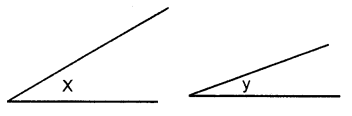Without measuring these angles, construct :
(i) ∠ABC = x + y
(ii) ∠ABC = 2x + y
(iii) ∠ABC = x + 2y
Solution: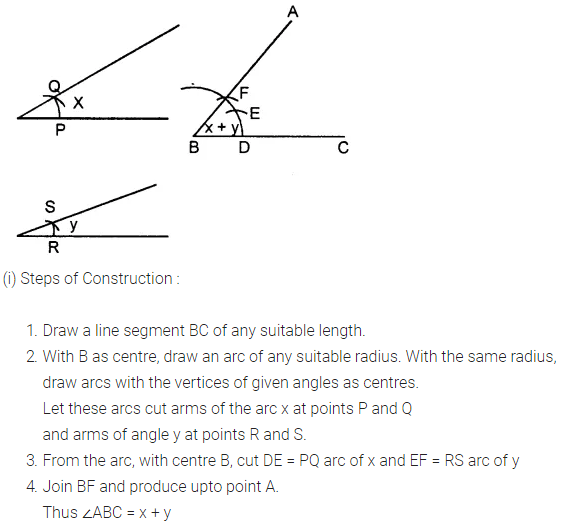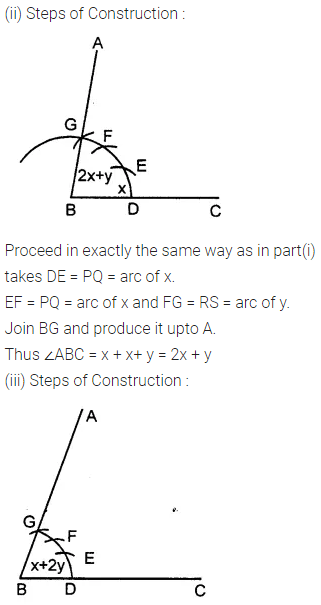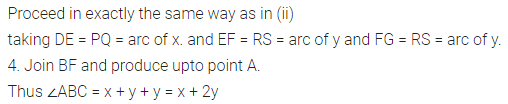Question 2.
Given below are the angles x, y and z.
Without measuring these angles construct :
(i) ∠ABC = x + y + z
(ii) ∠ABC = 2x + y + z
(iii) ∠ABC = x + 2y + zSolution: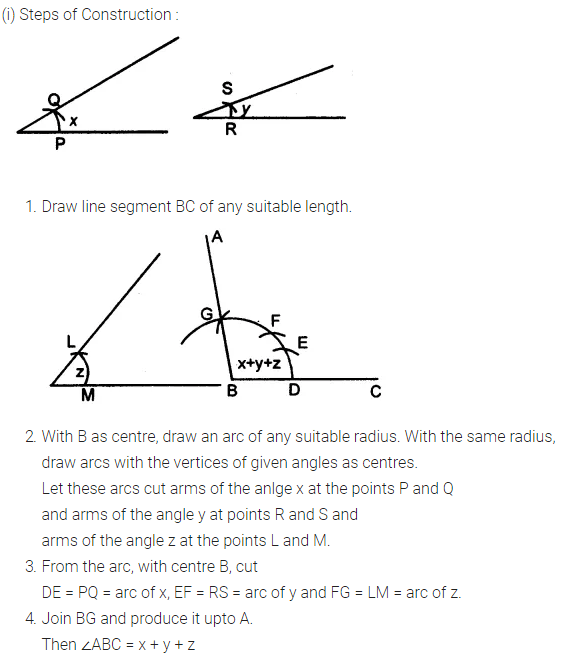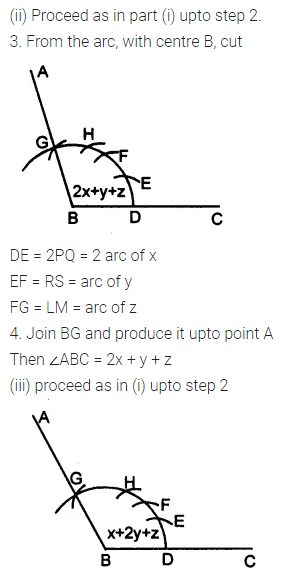Question 3.
Draw a line segment BC = 4 cm. Construct angle ABC = 60°.
Solution: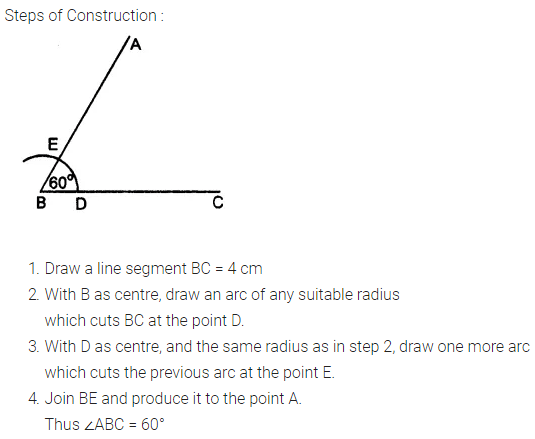Question 4.
Construct angle ABC = 45° in which BC = 5 cm and AB = 4.6 cm.
Solution: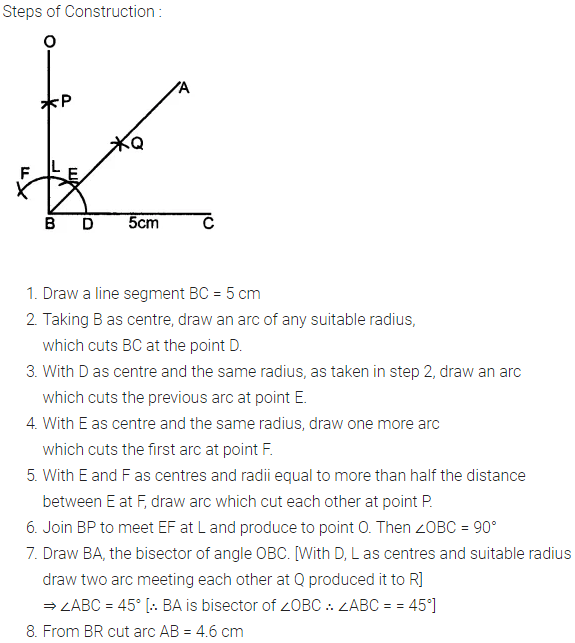Question 5.
Construct angle ABC = 90°. Draw BP, the bisector of angle ABC. State the measure of angle PBC.
Solution:Question 6.
6. Draw angle ABC of any suitable measure.
(i) Draw BP, the bisector of angle ABC.
(ii) Draw BR, the bisector of angle PBC and draw BQ, the bisector of angle ABP.
(iii) Are the angles ABQ, QBP, PBR and RBC equal?
(iv) Are the angles ABR and QBC equal ?
Solution: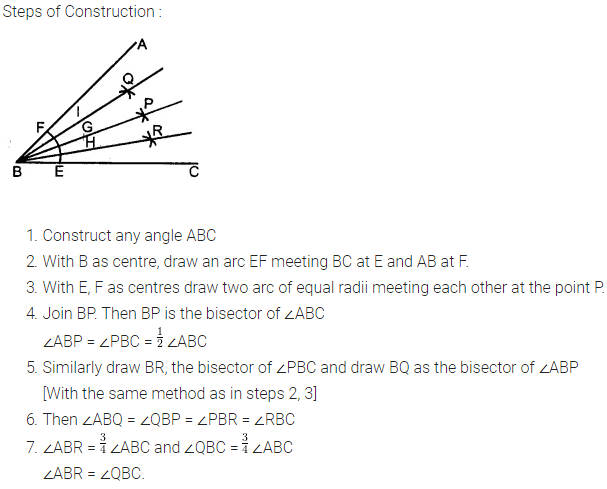### Constructions Exercise 18B – Selina Concise Mathematics Class 8 ICSE Solutions

Question 1.
Draw a line segment AB of length 5.3 cm. Using two different methods bisect AB.
Solution:Question 2.
Draw a line segment PQ = 4.8 cm. Construct the perpendicular bisector of PQ.
Solution:Question 3.
In each of the following, draw perpendicular through point P to the line segment AB :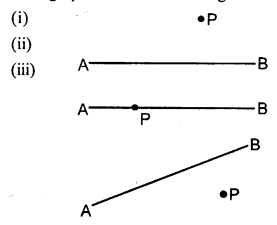Solution: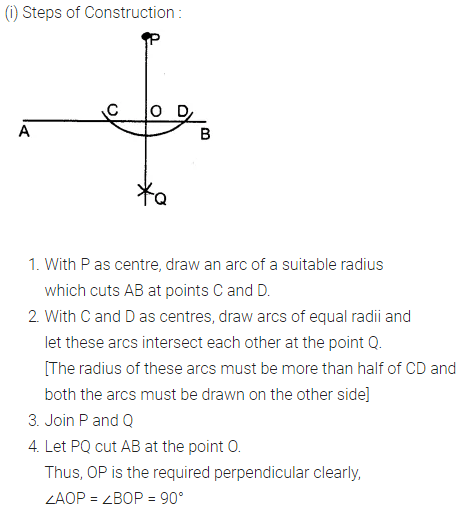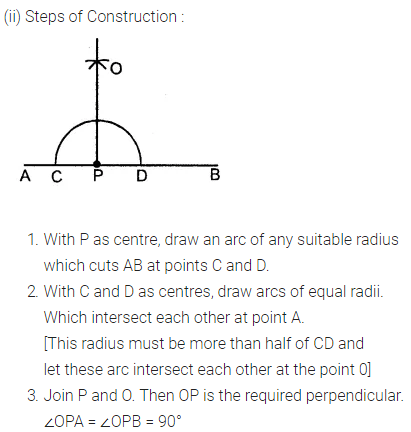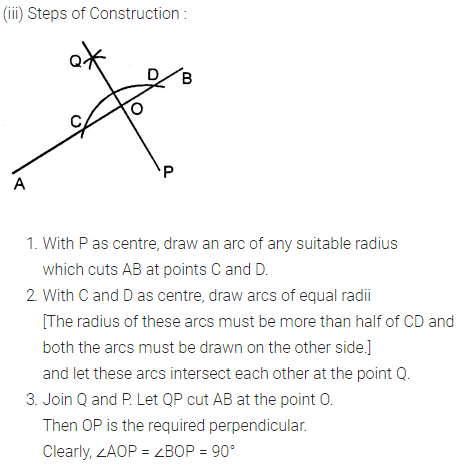Question 4.
Draw a line segment AB = 5.5 cm. Mark a point P, such that PA = 6 cm and PB = 4.8 cm. From the point P, draw a perpendicular to AB.
Solution: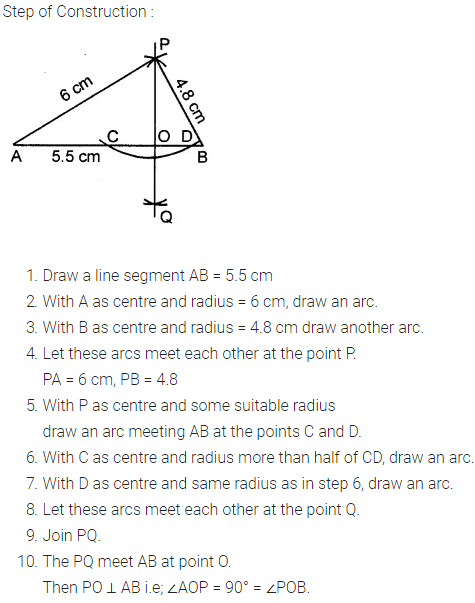Question 5.
Draw a line segment AB = 6.2 cm. Mark a point P in AB such that BP = 4 cm. Through point P draw perpendicular to AB.
Solution:### Constructions Exercise 18C – Selina Concise Mathematics Class 8 ICSE Solutions

Question 1.
Draw a line AB = 6 cm. Mark a point P any where outside the line AB. Through the point P, construct a line parallel to AB.
Solution:Question 2.
Draw a line MN = 5.8 cm. Locate a point A which is 4.5 cm from M and 5 cm from N. Through A draw a line parallel to line MN.
Solution: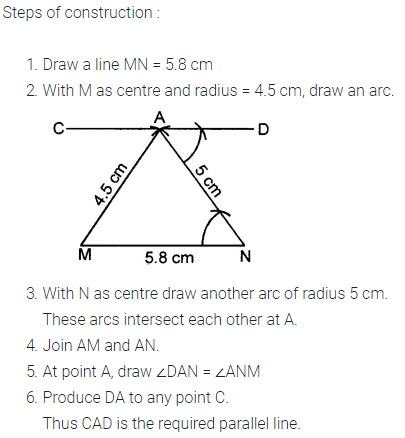Question 3.
Draw a straight line AB = 6.5 cm. Draw another line which is parallel to AB at a distance of 2.8 cm from it.
Solution: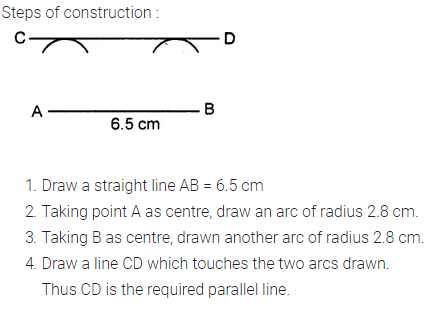Question 4.
Construct an angle PQR = 80°. Draw a line parallel to PQ at a distance of 3 cm from it and another line parallel to QR at a distance of 3.5 cm from it. Mark the point of intersection of these parallel lines as A.
Solution: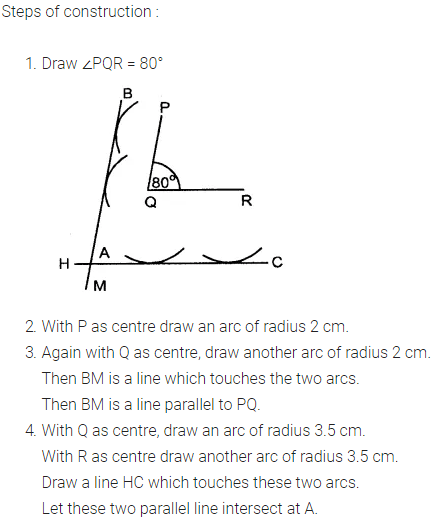Question 5.
Draw an angle ABC = 60°. Draw the bisector of it. Also draw a line parallel to BC a distance of 2.5 cm from it.
Let this parallel line meet AB at point P and angle bisector at point Q. Measure the length of BP and PQ. Is BP = PQ?
Solution: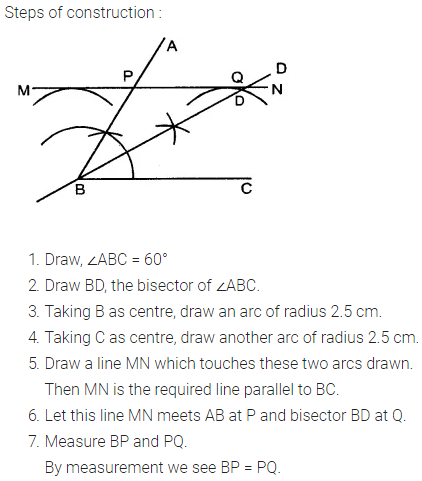Question 6.
Construct an angle ABC = 90°. Locate a point P which is 2.5 cm from AB and 3.2 cm from BC.
Solution:### Constructions Exercise 18D – Selina Concise Mathematics Class 8 ICSE Solutions

Question 1.
(i) AB = 4.3 cm, BC = 5.4, CD = 5 cm, DA = 4.8 cm and angle ABC = 75°.
(ii) AB = 6 cm, CD = 4.5 cm, BC = AD = 5 cm and ∠BCD = 60°.
(iii) AB = 8 cm, BC = 5.4 cm, AD = 6 cm, ∠A = 60° and ∠B = 75°.
(iv) AB = 5 cm, BC = 6.5 cm, CD =4.8 cm, ∠B = 75° and ∠C = 120°.
(v) AB = 6 cm = AC, BC = 4 cm, CD = 5 cm and AD = 4.5 cm.
(vi) AB = AD = 5cm, BD = 7 cm and BC = DC = 5.5 cm
Solution: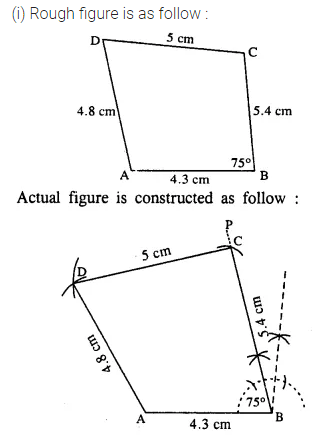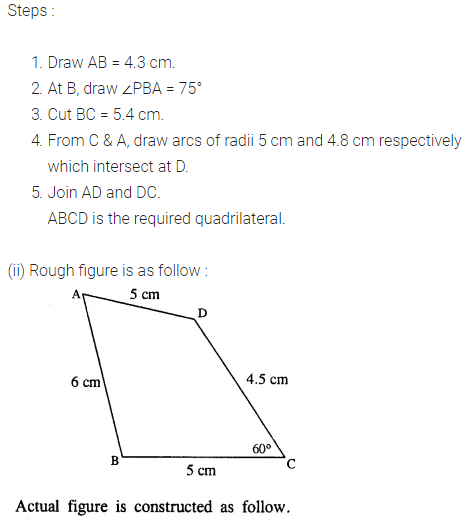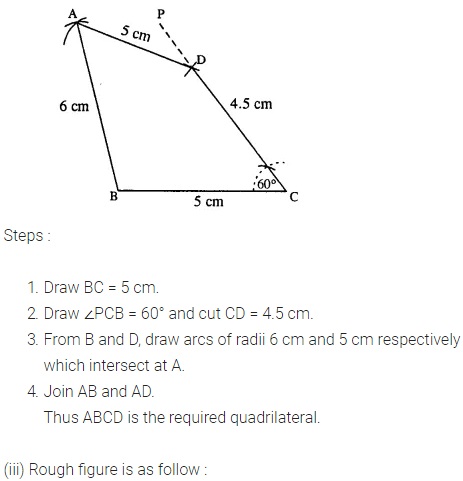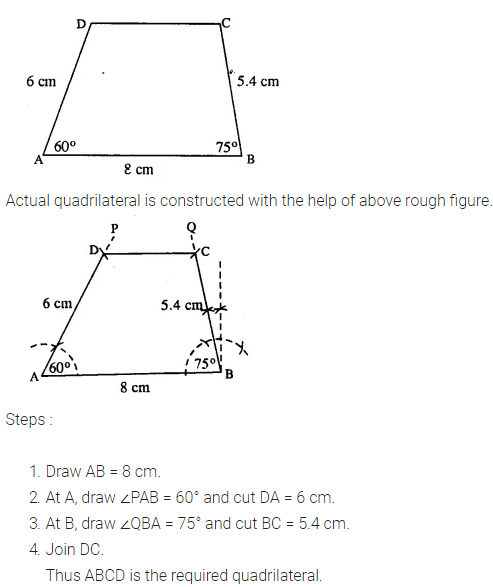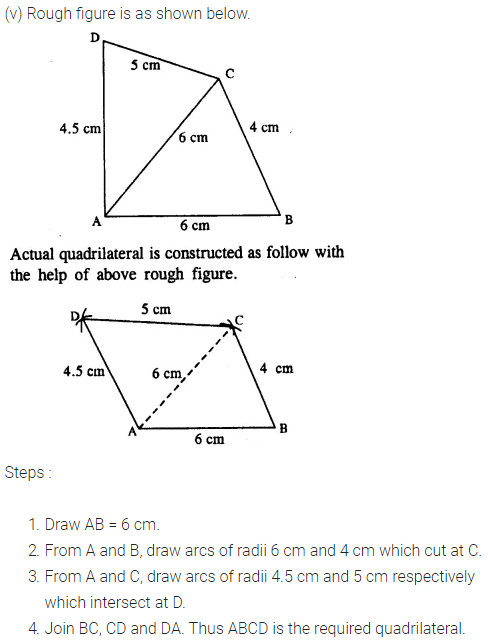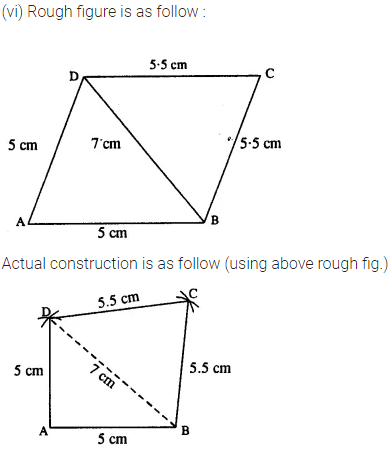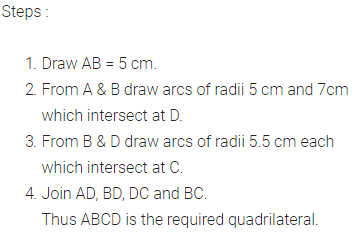Question 2.
Construct a parallelogram ABCD, if :
(i) AB = 3.6 cm, BC = 4.5 cm and ∠ABC = 120°.
(ii) BC = 4.5 cm, CD = 5.2 cm and ∠ADC = 75°.
(iii) AD = 4 cm, DC = 5 cm and diagonal BD = 7 cm.
(iv) AB = 5.8 cm, AD = 4.6 cm and diagonal AC = 7.5 cm.
(v) diagonal AC = 6.4 cm, diagonal BD = 5.6 cm and angle between the diagonals is 75°.
(vi) lengths of diagonals AC and BD are 6.3 cm and 7.0 cm respectively, and the angle between them is 45°.
(vii) lengths of diagonals AC and BD are 5.4 cm and 6.7 cm respectively and the angle between them is 60°.
Solution: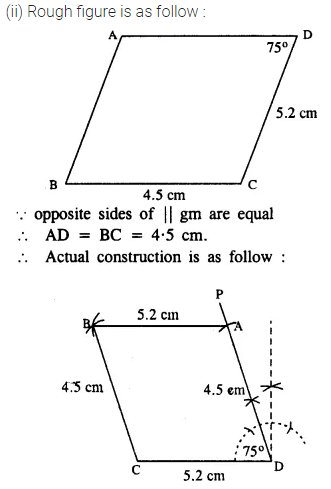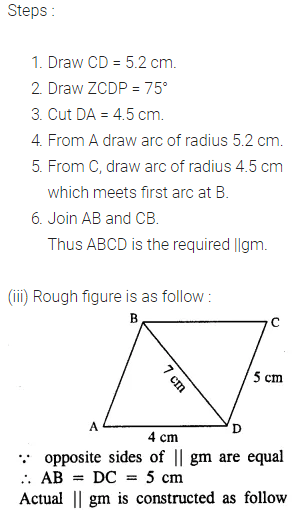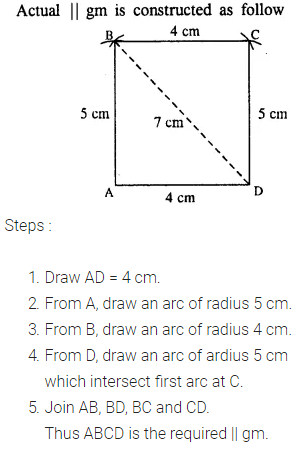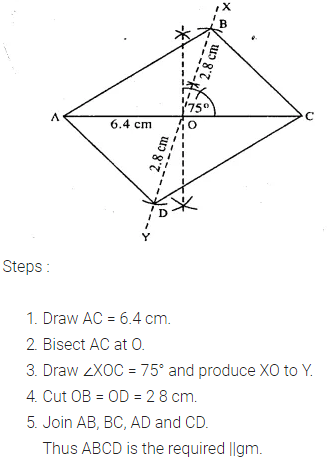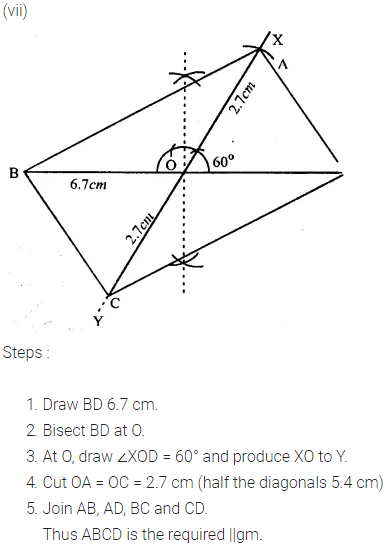Question 3.
Construct a rectangle ABCD ; if :
(i) AB = 4.5 cm and BC = 5.5 cm.
(ii) BC = 61 cm and CD = 6.8 cm.
(iii) AB = 5.0 cm and diagonal AC = 6.7 cm.
(iv) AD = 4.8 cm and diagonal AC = 6.4 cm.
(v) each diagonal is 6 cm and the angle between them is 45°.
(vi) each diagonal is 5.5 cm and the angle between them is 60°.
Solution: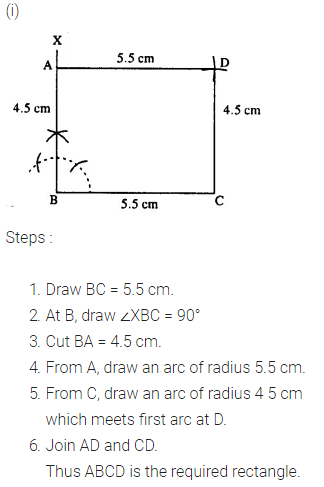Question 4.
Construct a rhombus ABCD, if ;
(i) AB = 4 cm and ∠B = 120°.
(ii) BC = 4.7 cm and ∠B = 75°.
(iii) CD = 5 cm and diagonal BD = 8.5 cm.
(iv) BC = 4.8cm, and diagonal AC = 7cm.
(v) diagonal AC = 6 cm and diagonal BD = 5.8 cm.
(vi) diagonal AC = 4.9 cm and diagonal BD = 6 cm.
(vii) diagonal AC = 6.6 cm and diagonal BD = 5.3 cm.
Solution: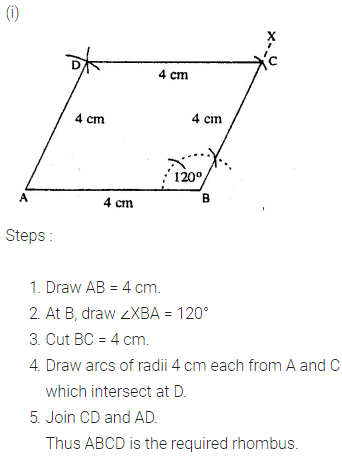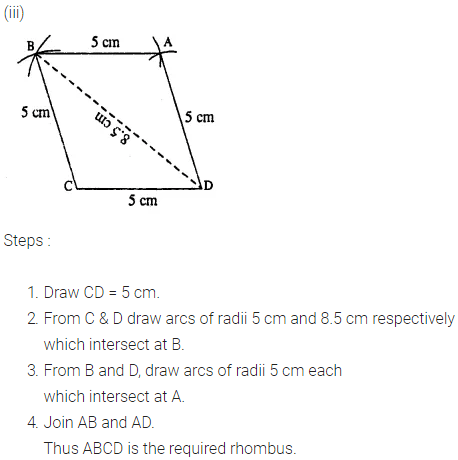Question 5.
Construct a square, if :
(i) its one side is 3.8 cm.
(ii) its each side is 4.3 cm.
(iii) one diagonal is 6.2 cm.
(iv) each diagonal is 5.7 cm.
Solution: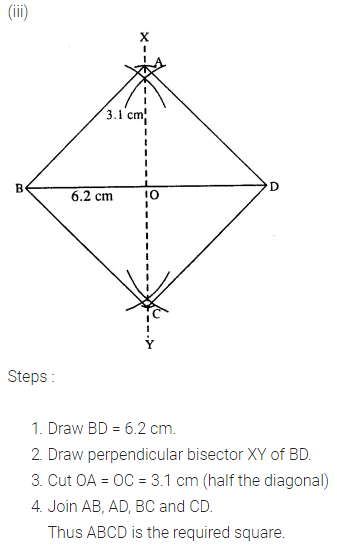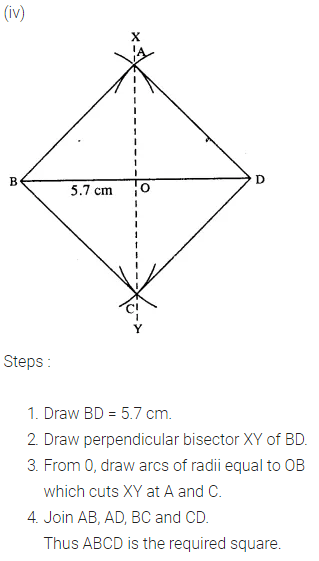Question 6.
Construct a quadrilateral ABCD in which ; ∠A = 120°, ∠B = 60°, AB = 4 cm, BC = 4.5 cm and CD = 5 cm.
Solution:Question 7.
Construct a quadrilateral ABCD, such that AB = BC = CD = 4.4 cm, ∠B = 90° and ∠C = 120°.
Solution: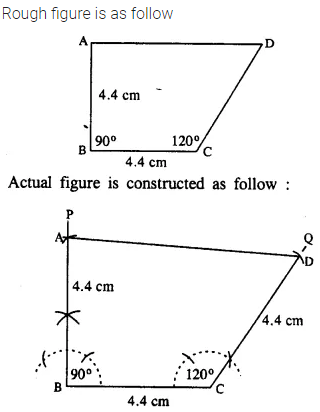Question 8.
Using ruler and compasses only, construct a parallelogram ABCD, in which : AB = 6 cm, AD = 3 cm and ∠DAB = 60°. In the same figure draw the bisector of angle DAB and let it meet DC at point P. Measure angle APB.
Solution:Question 9.
Draw a parallelogram ABCD, with AB = 6 cm, AD = 4.8 cm and ∠DAB = 45°. Draw the perpendicular bisector of side AD and let it meet AD at point P. Also draw the diagonals AC and BD ; and let they intersect at point O. Join O and P. Measure OP.
Solution: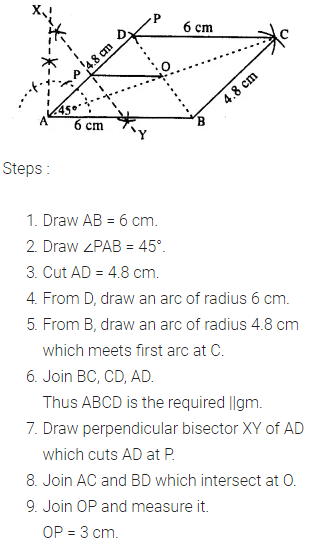Question 10.
Using ruler and compasses only, construct a rhombus whose diagonals are 8 cm and 6 cm. Measure the length of its one side.
Solution: